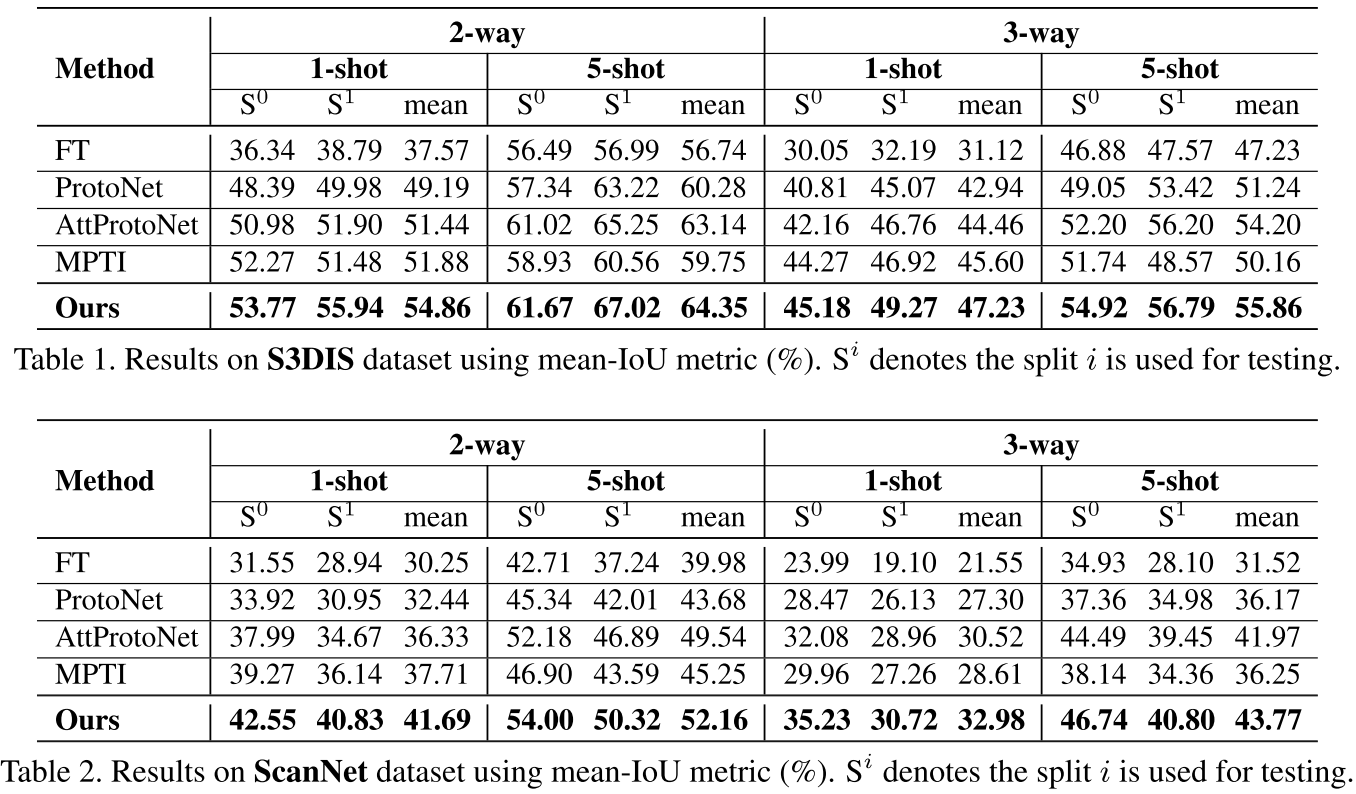• Prior Guided Feature Enrichment Network for Few-Shot Segmentation (TPAMI 2020)
• Few-Shot Segmentation Via Cycle-Consistent Transformer (NeurIPS 2021)
• Simpler is Better: Few-shot Semantic Segmentation with Classifier Weight Transformer (ICCV 2021)
• Few-Shot Segmentation Without Meta-Learning: A Good Transductive Inference Is All You Need? (CVPR 2021)
• Self-Guided and Cross-Guided Learning for Few-Shot Segmentation (CVPR 2021)
• Adaptive Prototype Learning and Allocation for Few-Shot Segmentation (CVPR 2021)
• Mining Latent Classes for Few-shot Segmentation (ICCV 2021)
• Few-Shot 3D Point Cloud Semantic Segmentation (CVPR 2021)

### Prior Guided Feature Enrichment Network for Few-Shot Segmentation

TPAMI 2020 | arxiv | github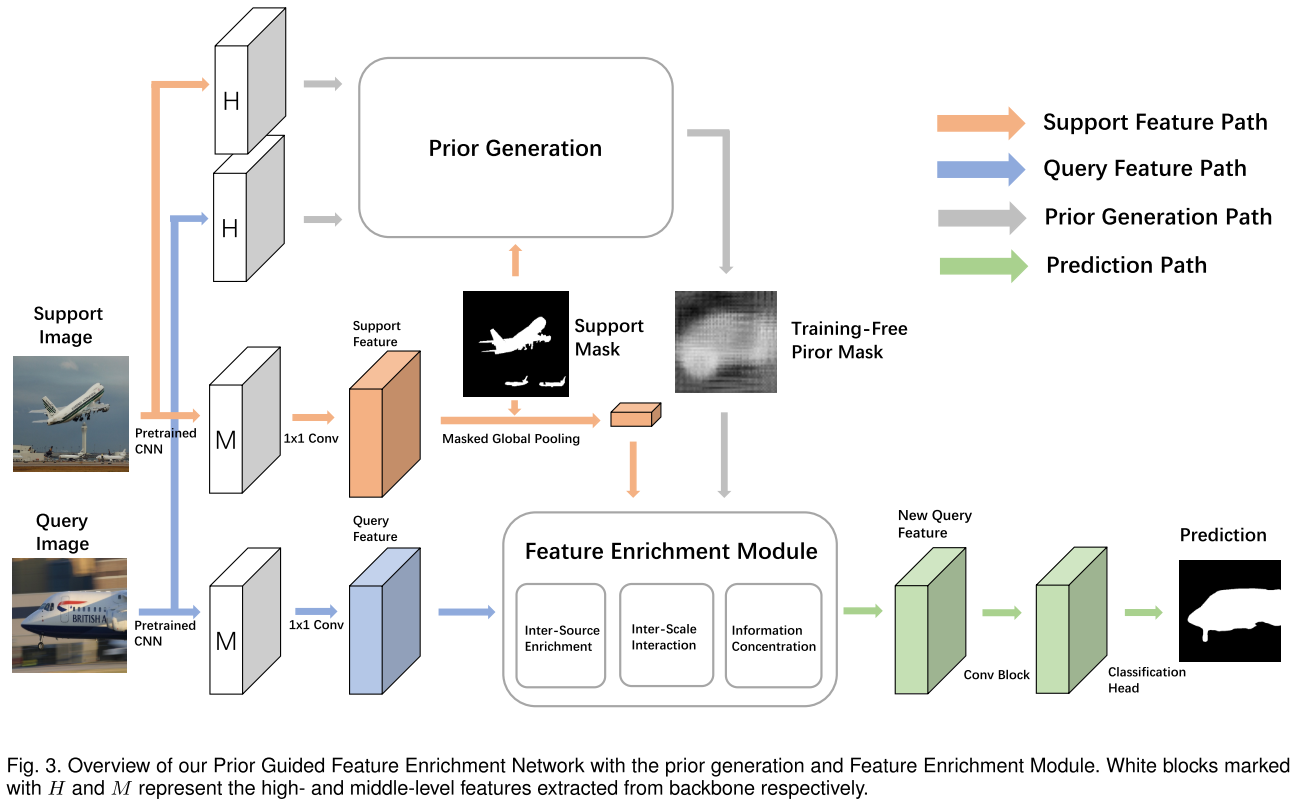prior generation：使用 high-level 特征（实践中是 conv5_x 的最后一层的输出）来生成 prior mask。在用 support mask 遮盖提取的 support 特征后，计算 support 和 query 特征的 pixel-wise cosine 相似度。对于 query 中的每个像素，取其和所有 support 像素的相似度中的最大值来得到 prior mask。之后 prior mask 经过 min-max normalization 来将所有值放缩到 [0, 1] 范围。如果 shot 数大于 1，将同一 query 特征和不同 support 特征得到的多张 prior masks 求平均来得到最终的 prior mask 输入到 FEM。

Feature Enrichment Module (FEM)：这一块看下图比较好理解，就是用多个 average pooling 来将 query 特征、support prototype 和 prior mask 的拼接产物放缩到不同大小，然后做 multi-scale 的信息交互。注意 query 特征和 support 特征是通过拼接 middle-level 特征（实践中是 conv3_x、conv_4x 的最后一层的输出）得到，另外如果 shot 数大于 1，直接取所有处理后的 support 特征的均值作为新的 support 特征。inter-scale interaction 的每个 scale 的产物也会过一个 3x3 + 1x1 的卷积层组成的分类头得到损失 $\mathcal{L}^i_1$，然后所有 scale 产物拼接后经过 1x1 卷积也用分类头得到损失 $\mathcal{L}_{2}$。最终的总损失为 $\mathcal{L} = \frac{\sigma}{n}\sum^n_{i=1} \mathcal{L}^i_1 + \mathcal{L}_{2}$，$\sigma$ 在所有实验中设为 1.0。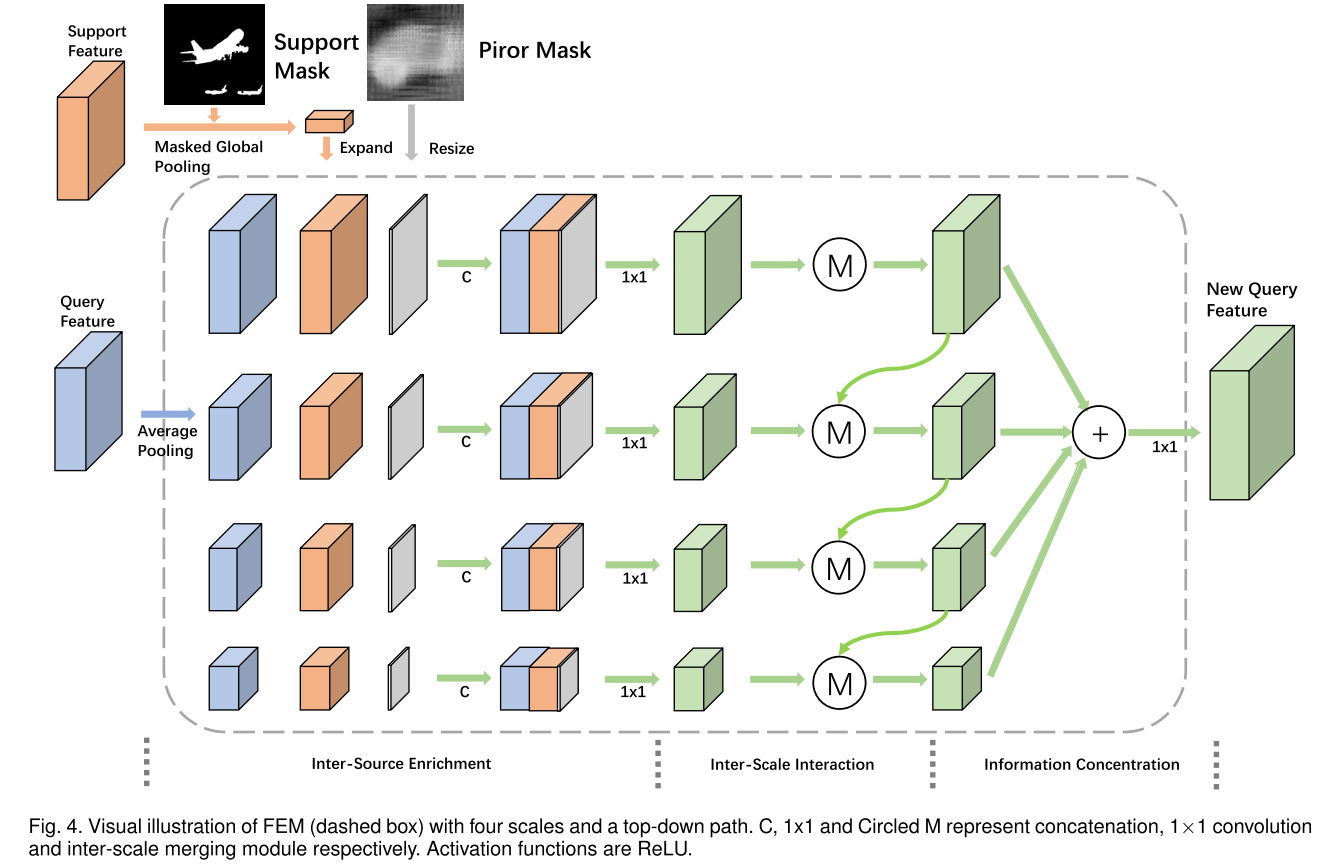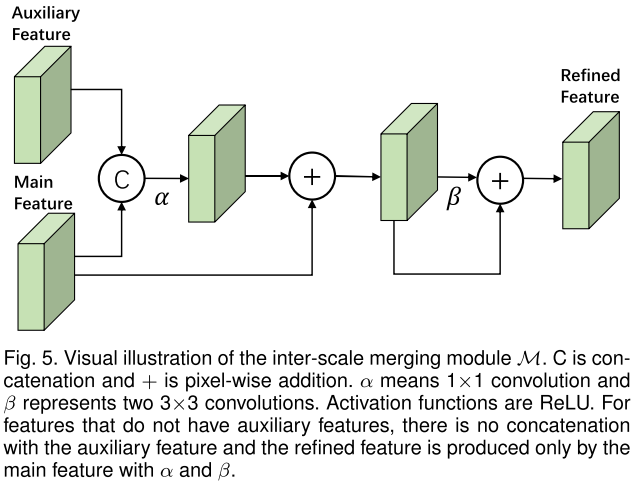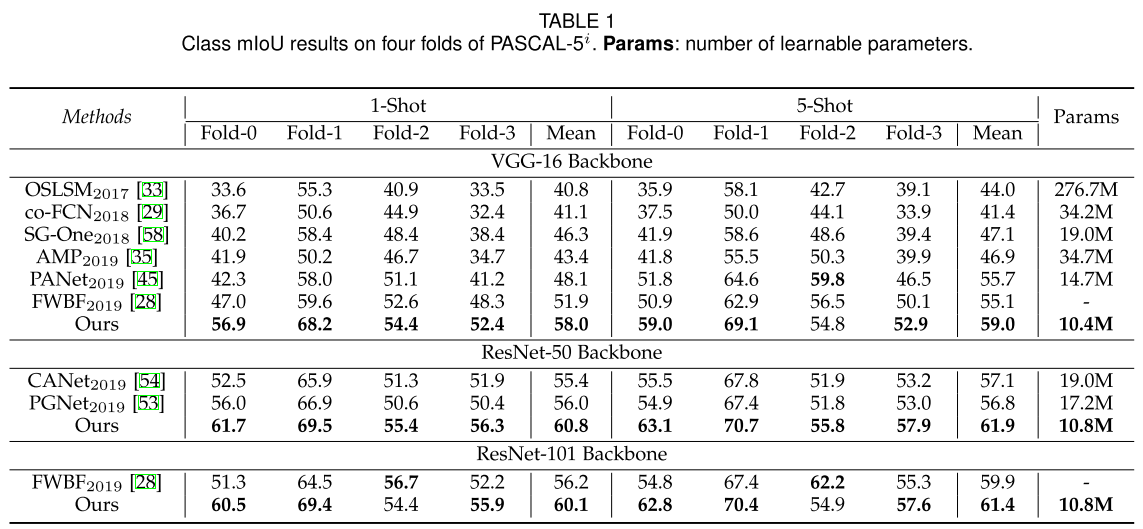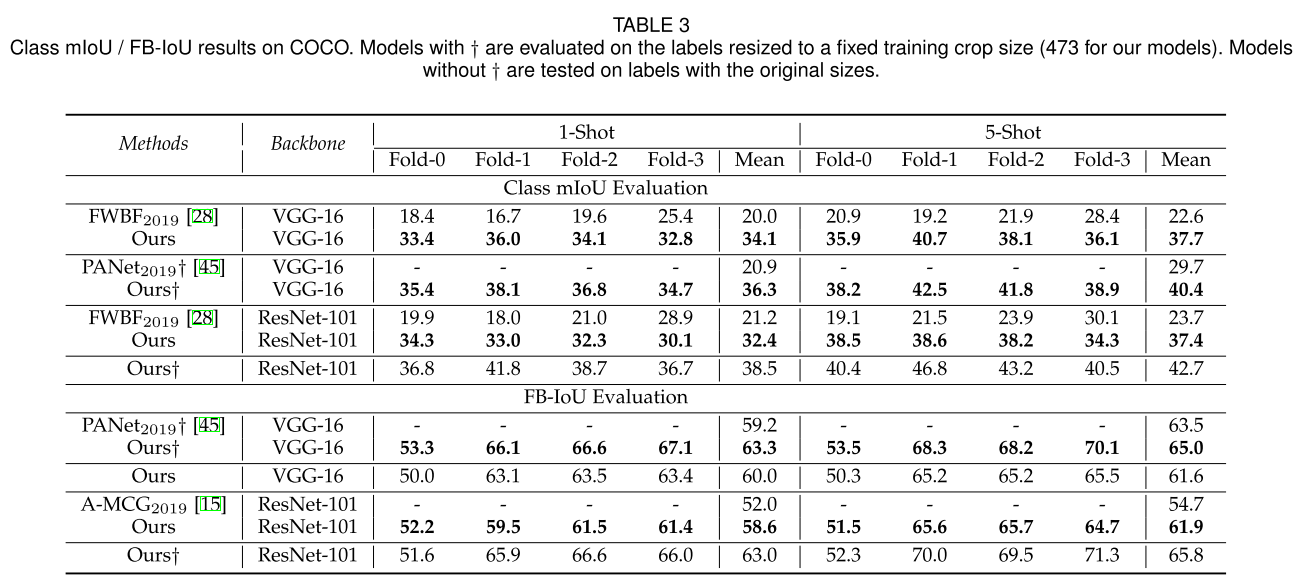### Few-Shot Segmentation Via Cycle-Consistent Transformer

NeurIPS 2021 | link | github（尚未包含代码）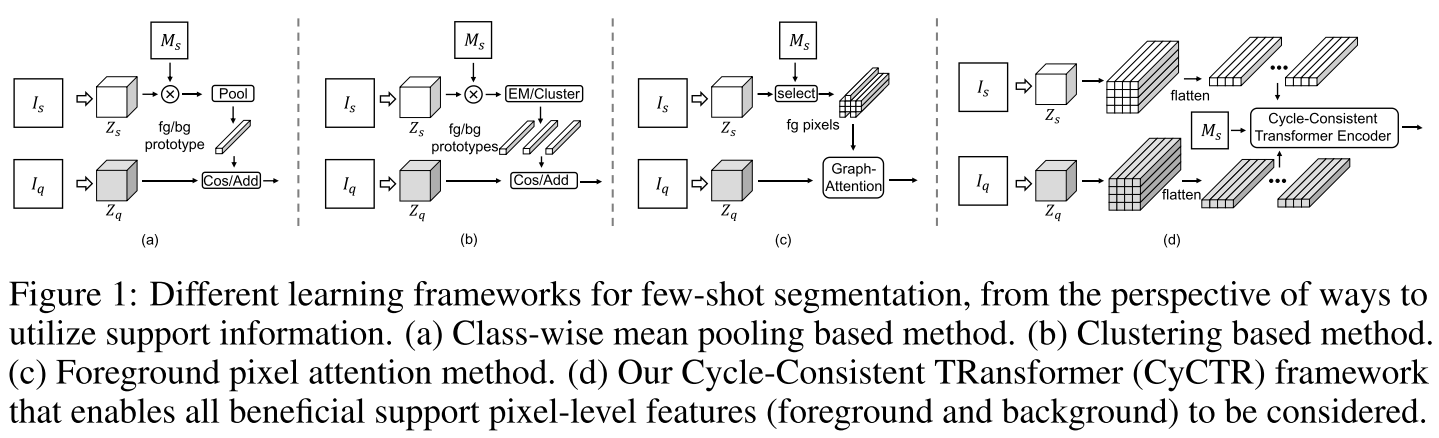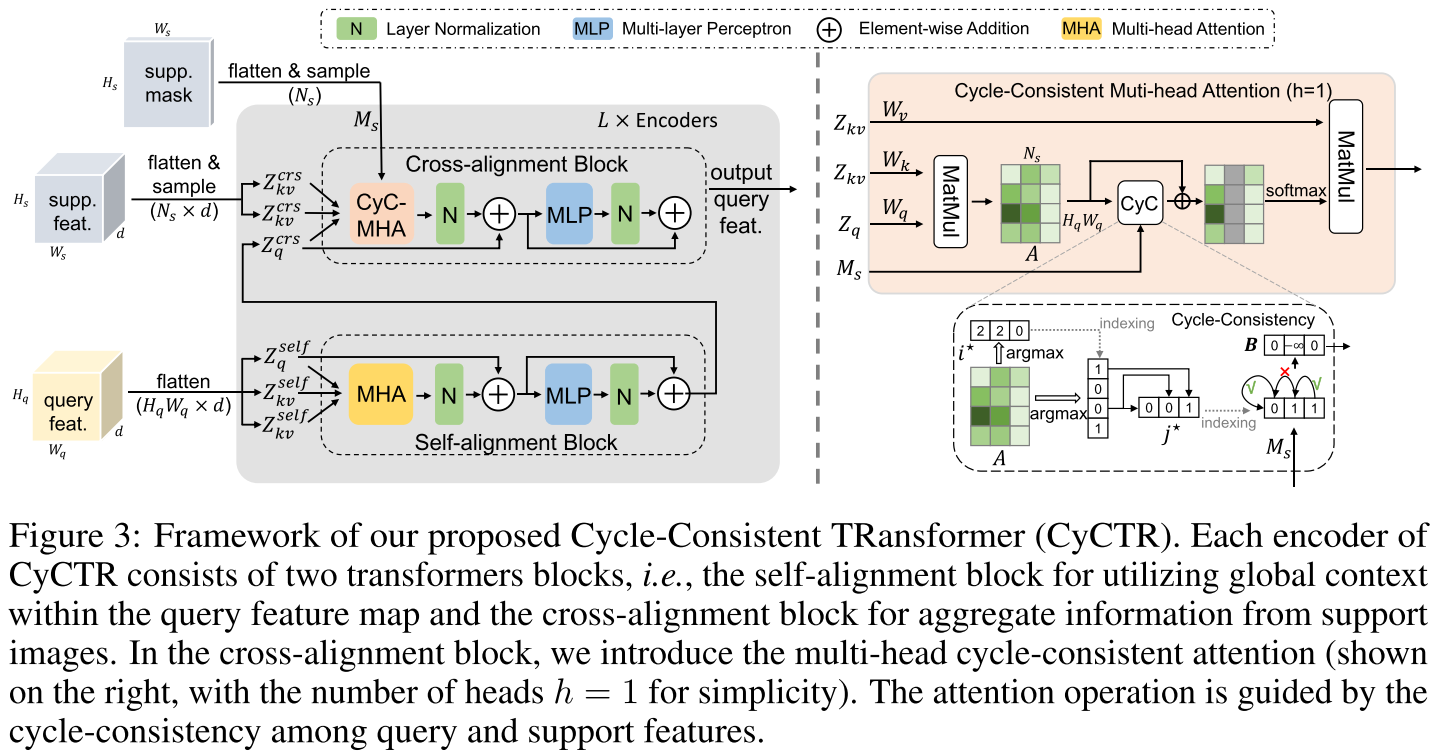$$B_{j}= \begin{cases}0, & \text { if } M_{s(j)}=M_{s\left(j^{\star}\right)} \\ -\infty, & \text { if } M_{s(j)} \neq M_{s\left(j^{\star}\right)} \end{cases}$$

$$\text{CyCAtten}(Q_i, K_i, V_i) = \text{softmax}(A_{i} + B)V$$

$$\text{PredAtten}(Q_r, V_r) = \sum^P_g\text{softmax}(A^{})_{(r,g)}V_{r+\triangle_{r,g}}$$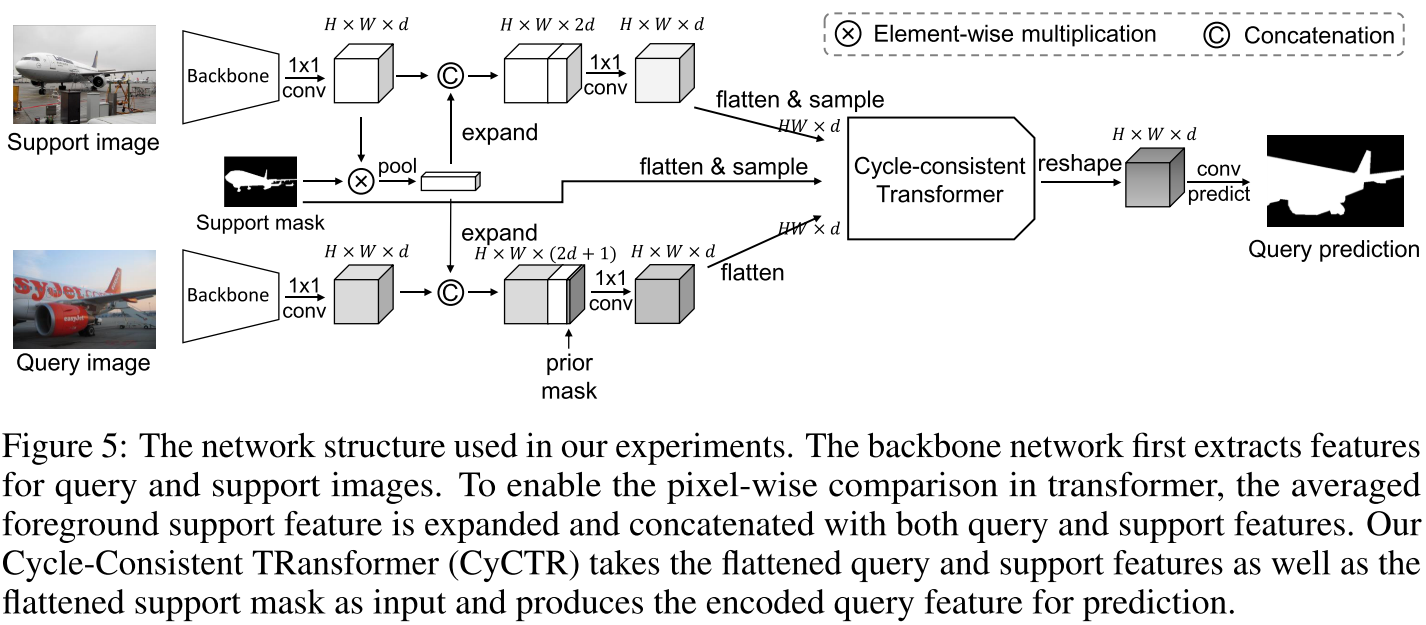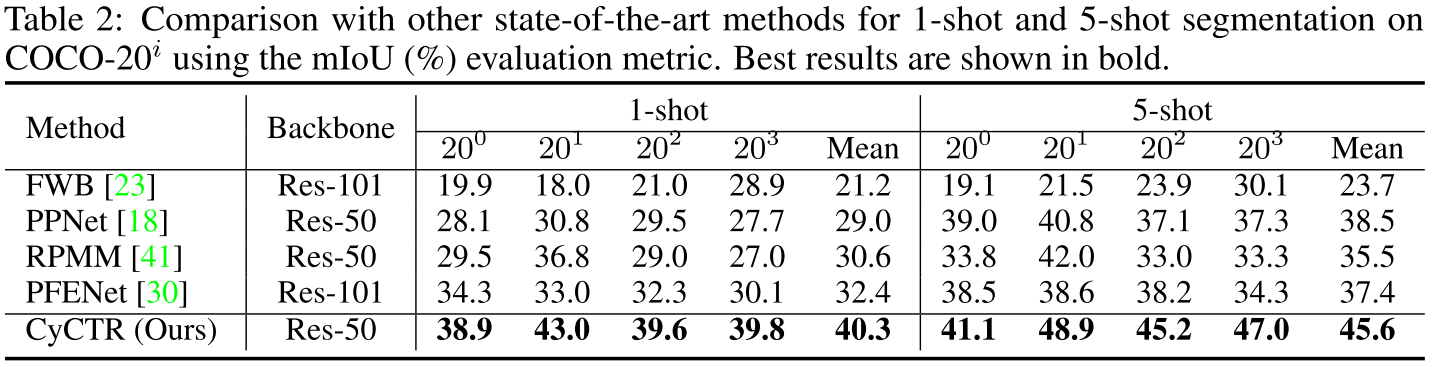### Simpler is Better: Few-shot Semantic Segmentation with Classifier Weight Transformer

ICCV 2021 | arxiv | github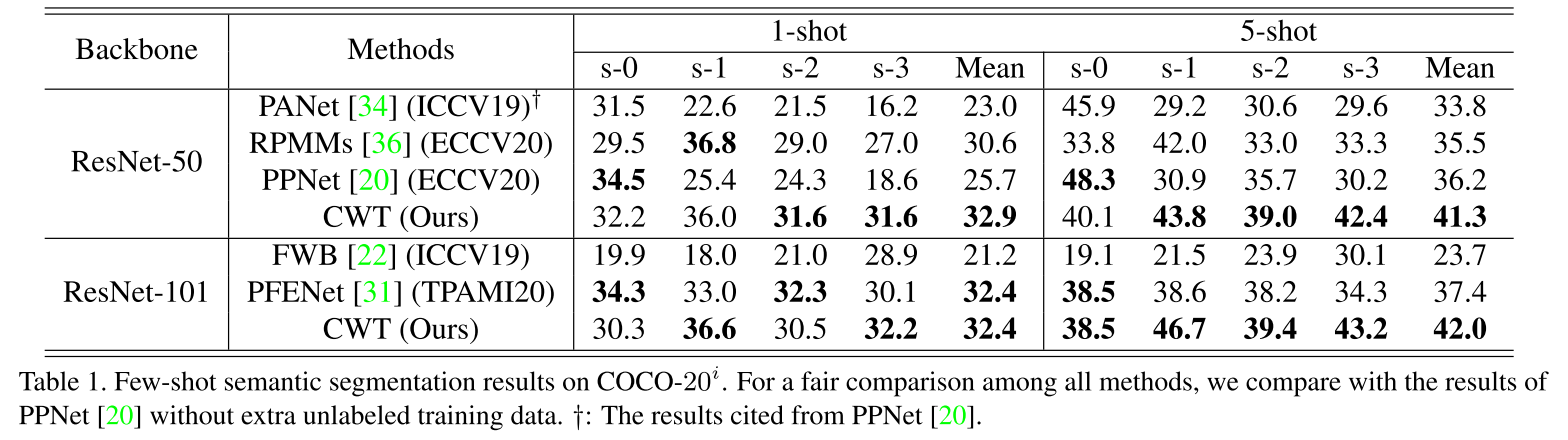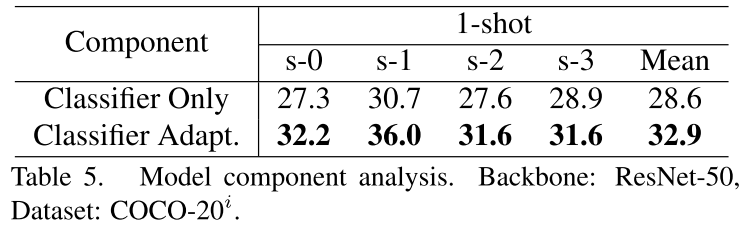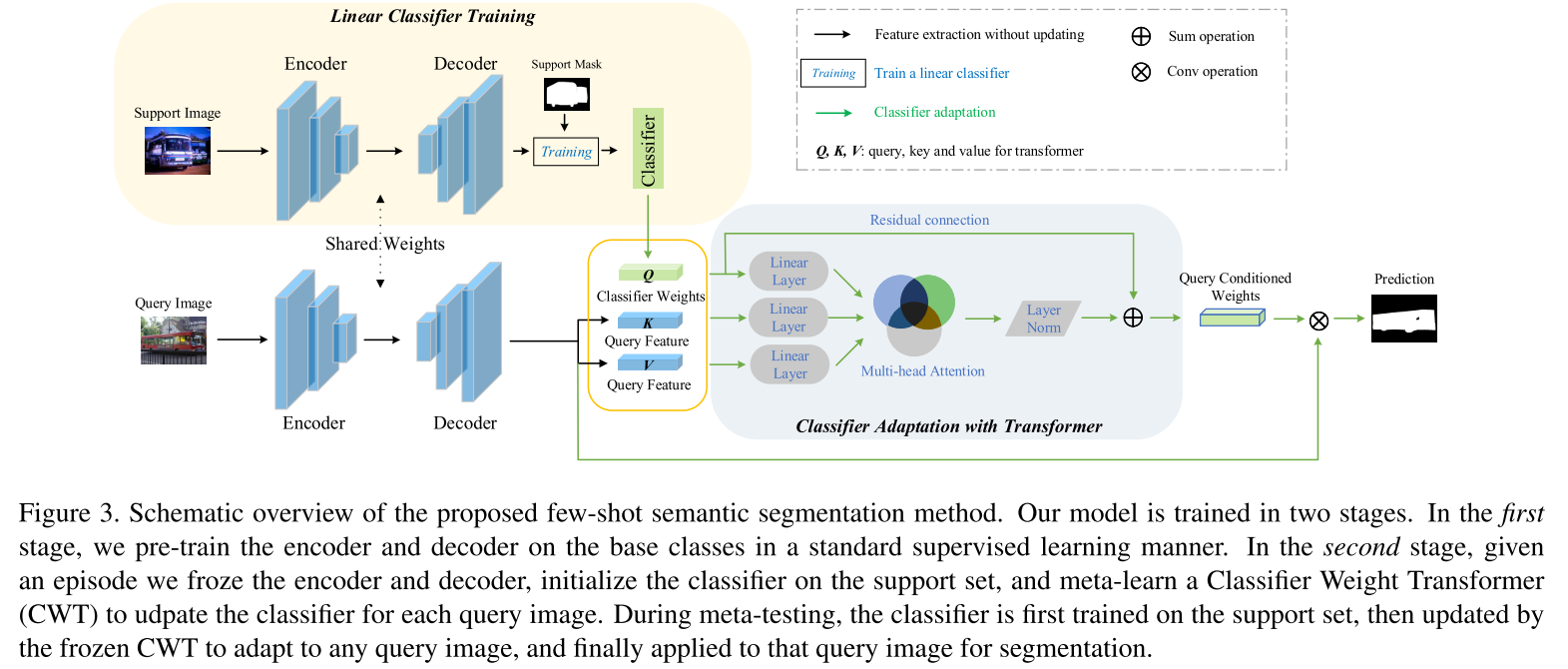$$\mathbf{w}^{*}=\mathbf{w}+\psi\left(\text{softmax}\left(\frac{\boldsymbol{w} \mathbf{W}_{q}\left(\boldsymbol{F} \mathbf{W}_{k}\right)^{\top}}{\sqrt{d_{a}}}\right)\left(\boldsymbol{F} \mathbf{W}_{v}\right)\right)$$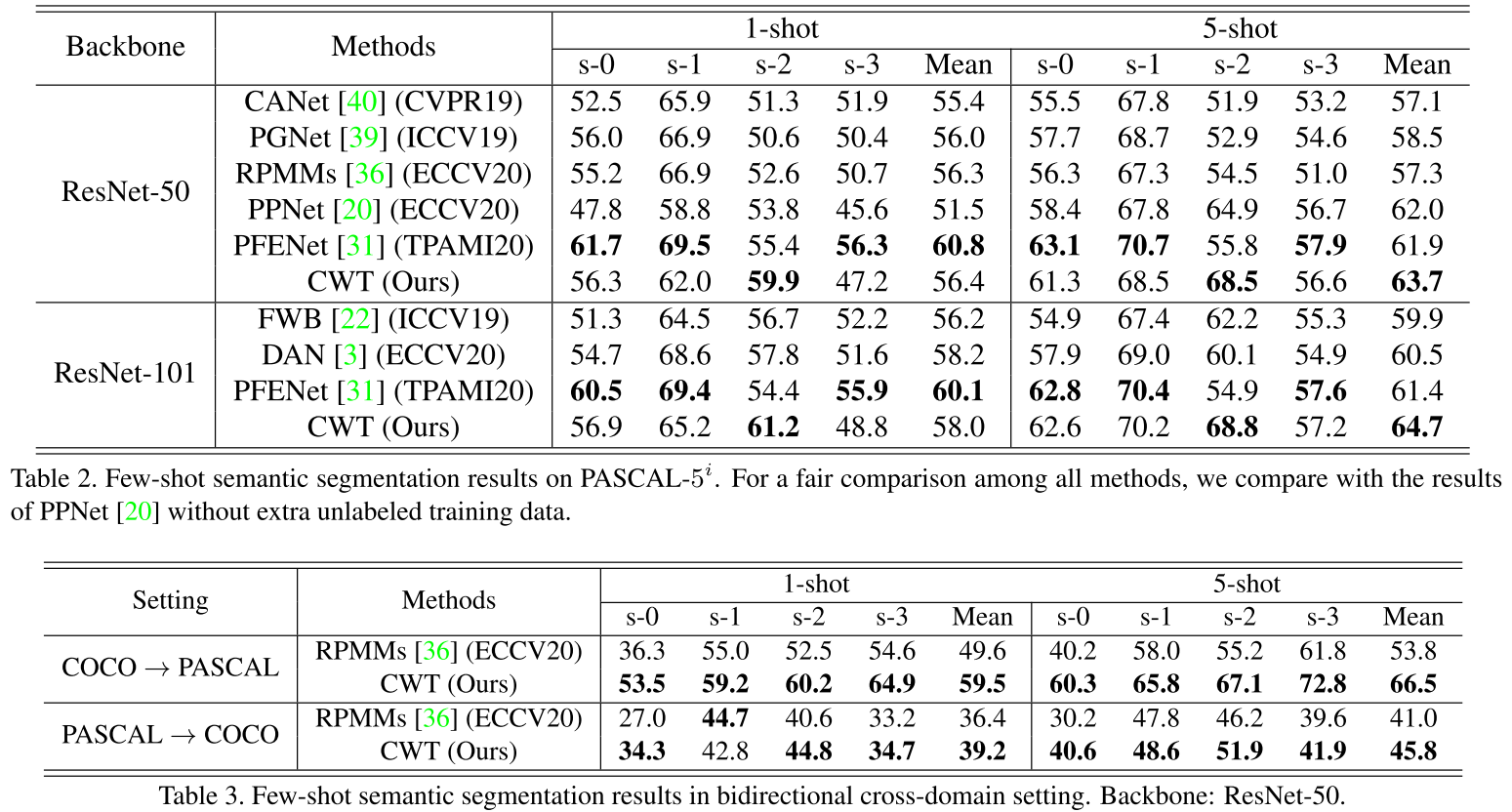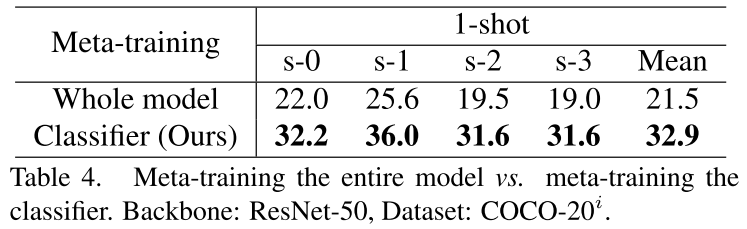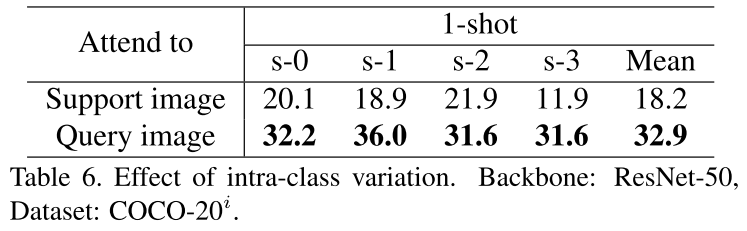### Few-Shot Segmentation Without Meta-Learning: A Good Transductive Inference Is All You Need?

CVPR 2021 | link | github

1. $\mathrm{CE}=-\frac{1}{K|\Psi|} \sum_{k=1}^{K} \sum_{j \in \Psi} \widetilde{y}_{k}(j)^{\top} \log \left(p_{k}(j)\right)$：标准的交叉熵 (cross-entropy, CE)，作用于 support 图像的有标签像素上。注意只使用这一项来进行优化通常导致过拟合于 support set，尤其是 1-shot 设置时；
2. $\mathcal{H}=-\frac{1}{|\Psi|} \sum_{j \in \Psi} p_{\mathcal{Q}}(j)^{\top} \log \left(p_{\mathcal{Q}}(j)\right)$：香农熵 (Shannon entropy)，作用于 query 图像像素的预测上，来使模型对 query 图像的预测更加有信心。直观来说，这一项让线性分类器的决策边界推向 query 特征空间的低密度区域。在对最初置信度较低的区域的预测有帮助的同时，仅仅将这项加入到损失中并不能解决 CE 导致的问题，甚至可能使表现进一步恶化，如下图 Figure 1 所示；
3. $\mathcal{D}_{\mathrm{KL}}=\widehat{p}_{\mathcal{Q}}^{\top} \log \left(\frac{\widehat{p}_{\mathcal{Q}}}{\pi}\right), \widehat{p}_{\mathcal{Q}}=\frac{1}{|\Psi|} \sum_{j \in \Psi} p_{\mathcal{Q}}(j)$：KL 散度，鼓励模型预测的背景/前景 (B/F) 比例接近于一个参数 $\pi \in [0, 1]^2$。论文指出这一项在损失中占关键位置，首先当参数 $\pi$ 与 query 图像的精确 B/F 比例不匹配时，该项有助于避免因 $\mathrm{CE}$ 和 $\mathcal{H}$ 最小化而导致的退化解；而如果能够准确估计 query 图像中的 B/F 比例（即有这个先验知识可供使用时），该项可以大幅提高方法整体的性能。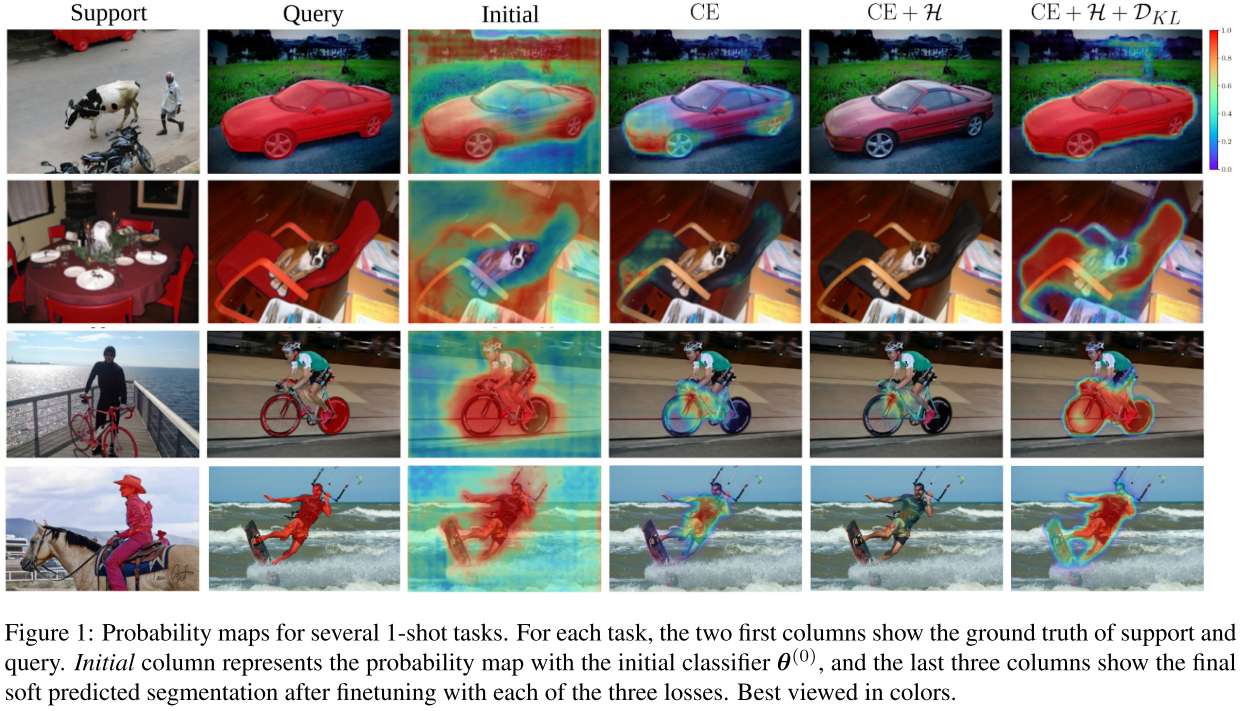B/F 比例 $\pi$ 的联合估计：当没有先验知识时，RePRI 使用 $\widehat{p}_{\mathcal{Q}}$ 来联合学习 $\pi$ 和分类器的参数，这时 $\mathcal{D}_{\mathrm{KL}}$ 可以被视为 self-regularization 来防止模型的 marginal distribution 发生偏移。具体实现中，只在初始化后在之后的某一轮迭代 $t_{\pi}$ 更新一次 $\pi$ 即可，即

$$\pi^{(t)}= \begin{cases}\widehat{p}_{\mathcal{Q}}^{(0)} & 0 \leq t \leq t_{\pi} \\ \hat{p}_{\mathcal{Q}}^{\left(t_{\pi}\right)} & t>t_{\pi}\end{cases}$$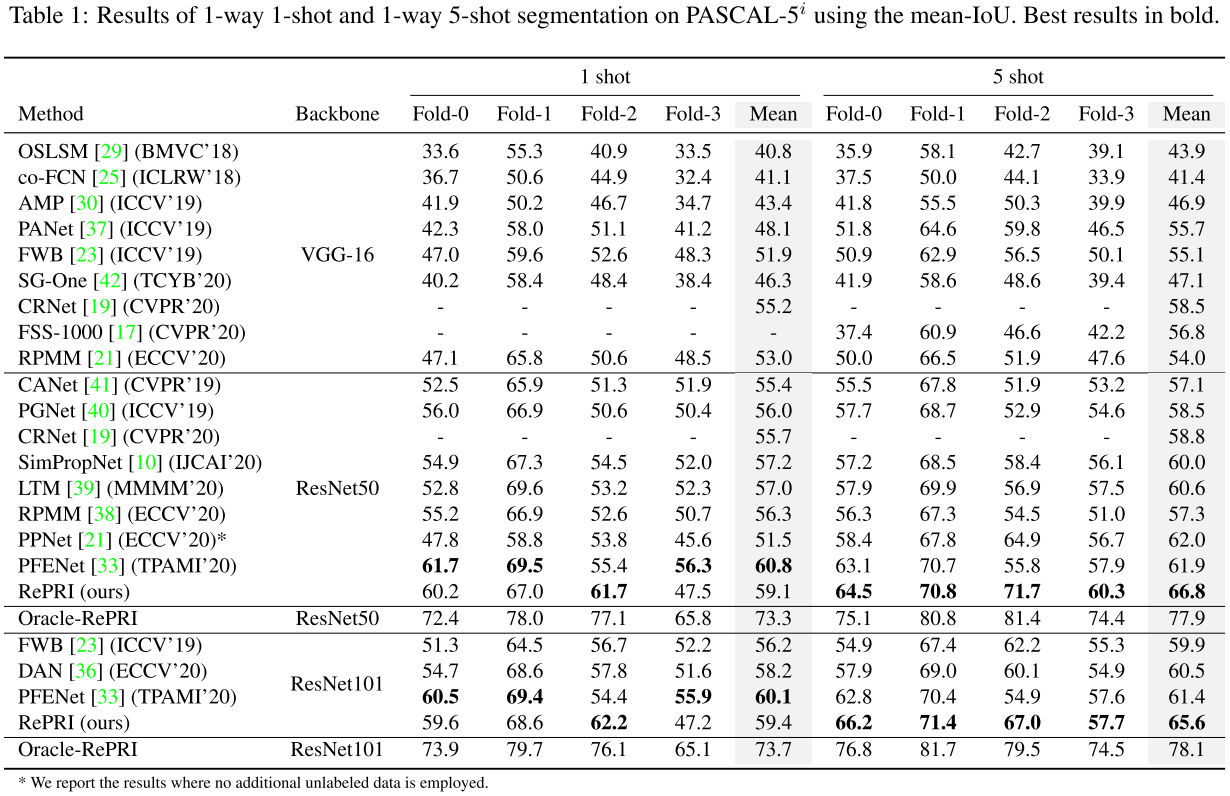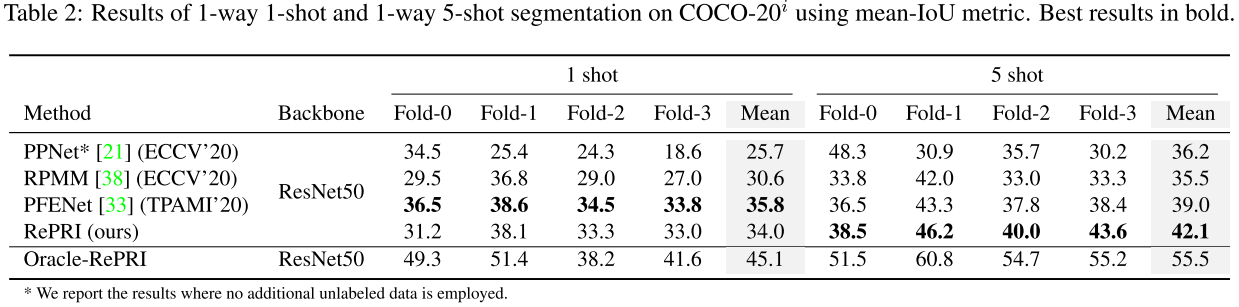### Self-Guided and Cross-Guided Learning for Few-Shot Segmentation

CVPR 2021 | link | github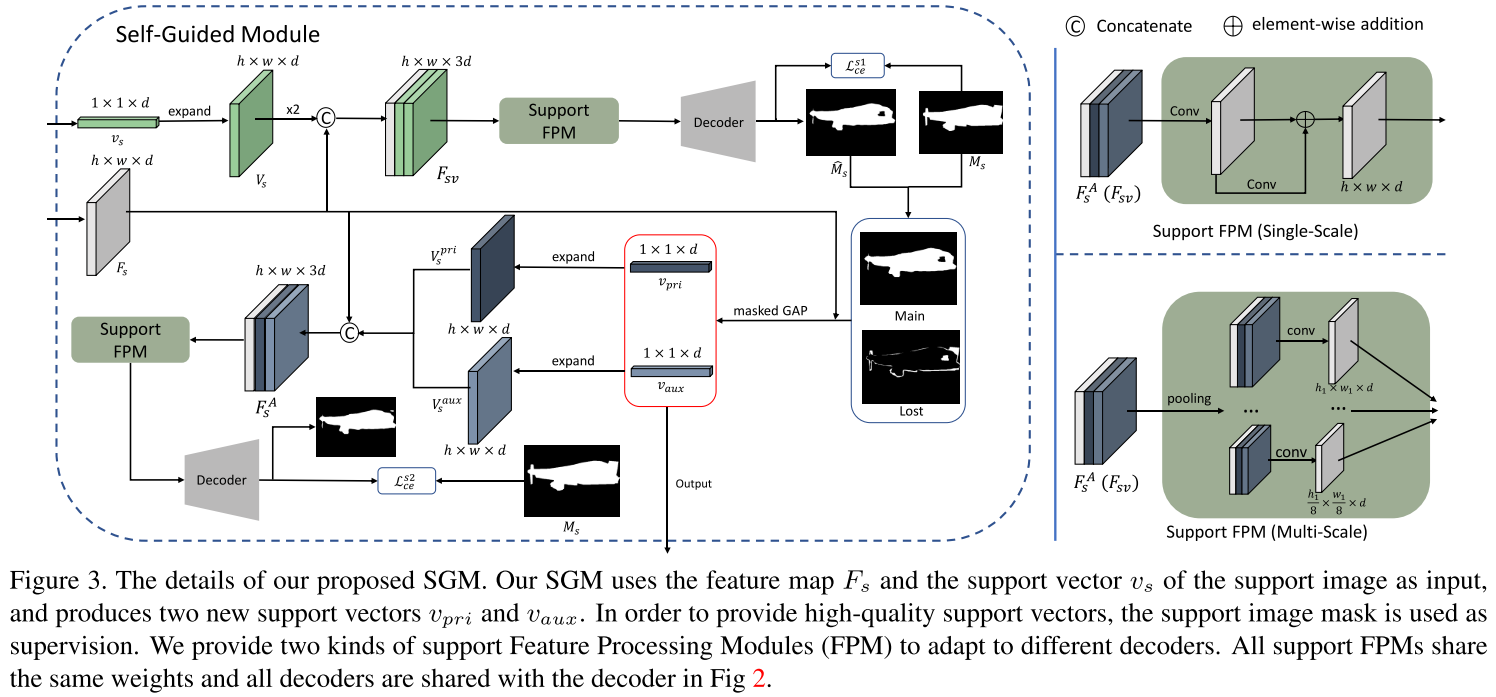Self-Guided Learning on Support Set：这里首先以 1-shot 为例。用 masked GAP 获得的初始 support 向量 $\mathcal{v}_s$ 被扩展成和 support 特征图 $F_s$ 同样大小的 $V_s$，然后拼接得到新的特征图 $F_{sv} = \text{Concat}([F_s, V_s, V_s])$。通过将 $F_{sv}$ 输入到 support FPM 和 decoder，可以得到support 图像的概率图 $P_{s1} = \text{softmax}(\mathcal{D}(FPM_s(F_{sv})))$，其中 $\mathcal{D}(\cdot)$ 指 decoder。由于 SCL 是一个即插即用的模块，因此 support FPM 和 decoder 的设计遵循所选择的小样本分割 baseline。本文的实验部分选择插入到两种 baseline 中：(1) CANet (CVPR 2019)，其 decoder 是 single-scale 结构的，因此 SCL 也采用 single-scale support FPM；(2) PFENet (TPAMI 2020)，其 decoder 是 multi-scale 结构的，因此 SCL 也采用 multi-scale support FPM。single-scale 和 multi-scale 的 support FPM 结构如上图右边所示。

$$\mathcal{L}_{c e}^{s 1}=-\frac{1}{h w} \sum_{i=1}^{h w} \sum_{c_{j} \in{0,1}}\left[M_{s}(i)=c_{j}\right] \log \left(P_{s 1}^{c_{j}}(i)\right)$$

$$\mathcal{L}_{c e}^{s 2}=-\frac{1}{h w} \sum_{i=1}^{h w} \sum_{c_{j} \in{0,1}}\left[M_{s}(i)=c_{j}\right] \log \left(P_{s 2}^{c_{j}}(i)\right)$$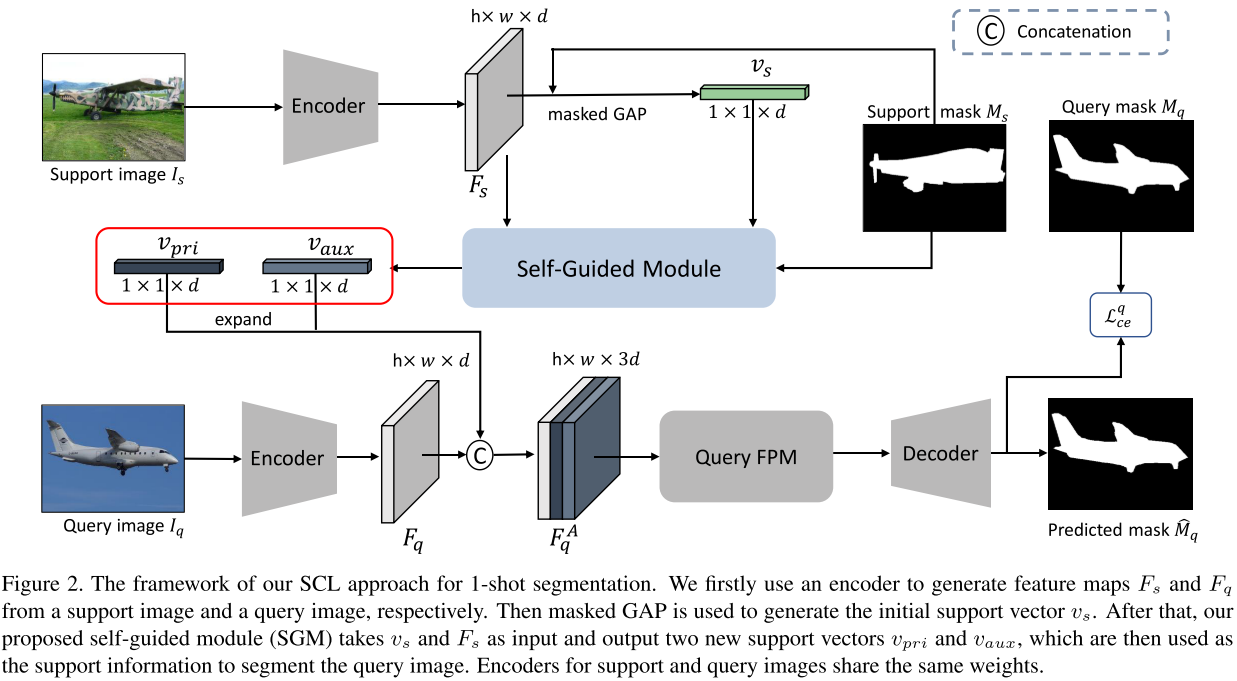Training on Query Set：对于 query 的特征图 $F_q$，流程为 $F^A_{q} = \text{Concat}([F_q, V^{pri}_q, V^{aux}_q])$，其中 $V^{pri}_q$ 和 $V^{aux}_q$ 是 $\mathcal{v}_{pri}$ 和 $\mathcal{v}_{aux}$ 扩展大小得到；$P_{q} = \text{softmax}(\mathcal{D}(FPM_q(F^A_{q})))$，注意这里有一个单独的 query FPM $FPM_q$ 而非 $FPM_s$。同样计算交叉熵得到 $\mathcal{L}_{c e}^{q}$：

$$\mathcal{L}_{c e}^{q}=-\frac{1}{h w} \sum_{i=1}^{h w} \sum_{c_{j} \in{0,1}}\left[M_{q}(i)=c_{j}\right] \log \left(P_{q}^{c_{j}}(i)\right)$$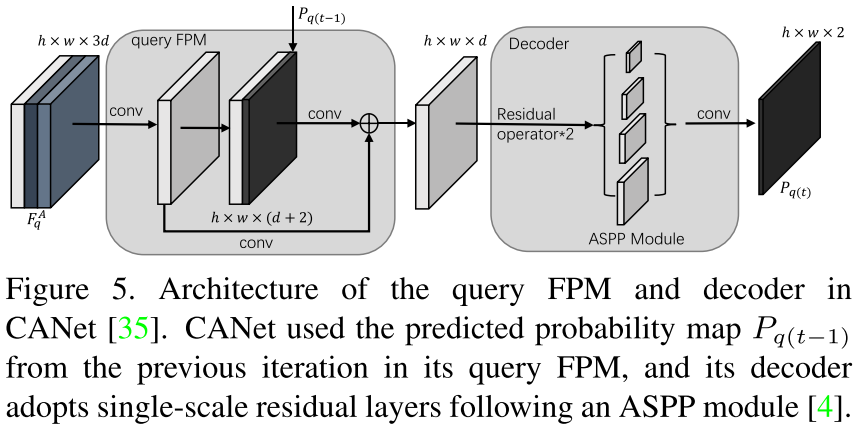Cross-Guided Multiple Shot Learning：当 shot 数 > 1 时，对于第 k 张 support 图像，首先将其作为 support 图像，将所有 K 张 support 图像作为 query 图像来输入到所提出的面向 1-shot 的模型中。对于第 i 张 support 图像，得到在第 k 张图像的支持下的预测 mask $\hat{M}_{s}^{i \mid k}$。因为第 i 张 support 图像的 ground-truth mask $M_s^i$ 是可得的，因此可以用预测和 ground-truth 的 masks 的 IOU 来计算一个置信度：

$$U_{s}^{k}=\frac{1}{K} \sum_{i=1}^{K} \text{IOU}\left(\hat{M}_{s}^{i \mid k}, M_{s}^{i}\right)$$

$$\hat{P}_{q}=\text{softmax}\left(\frac{1}{K} \sum_{k=1}^{K} U_{s}^{k} \mathcal{G}\left(I_{q} \mid I_{s}^{k}\right)\right)$$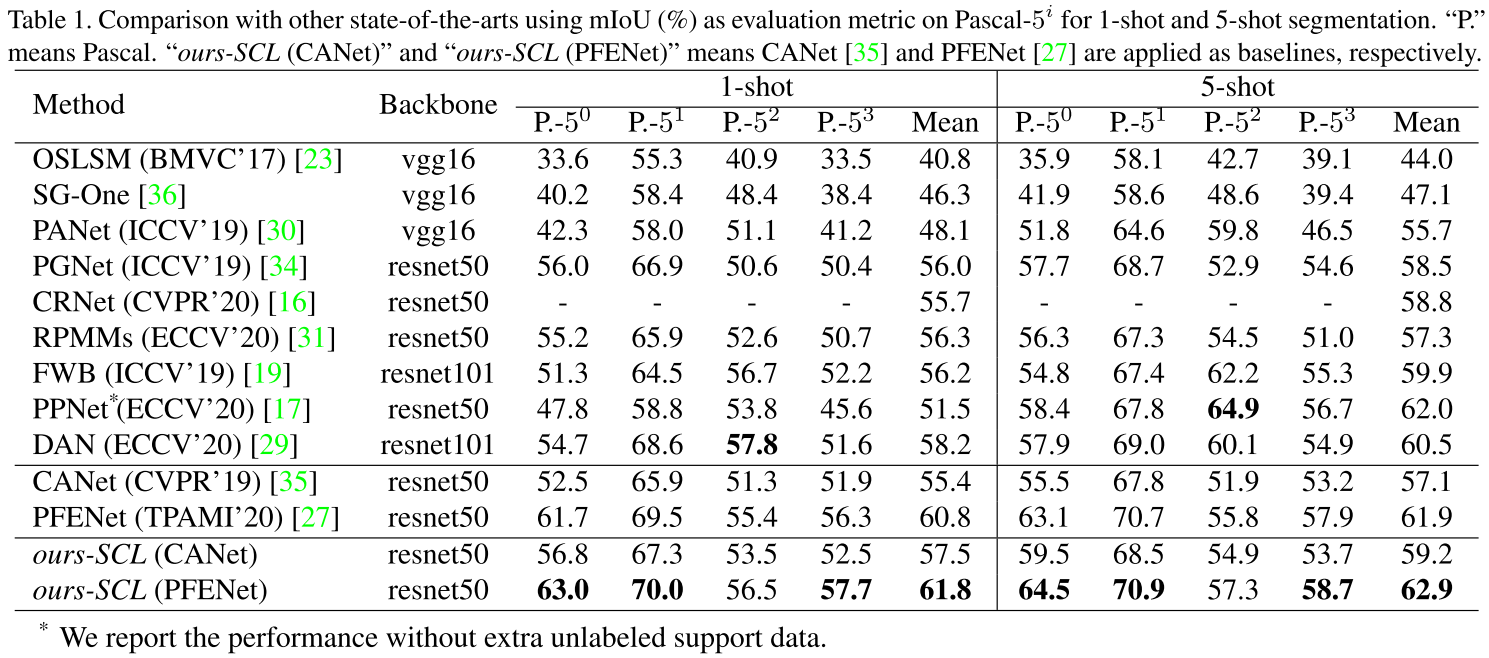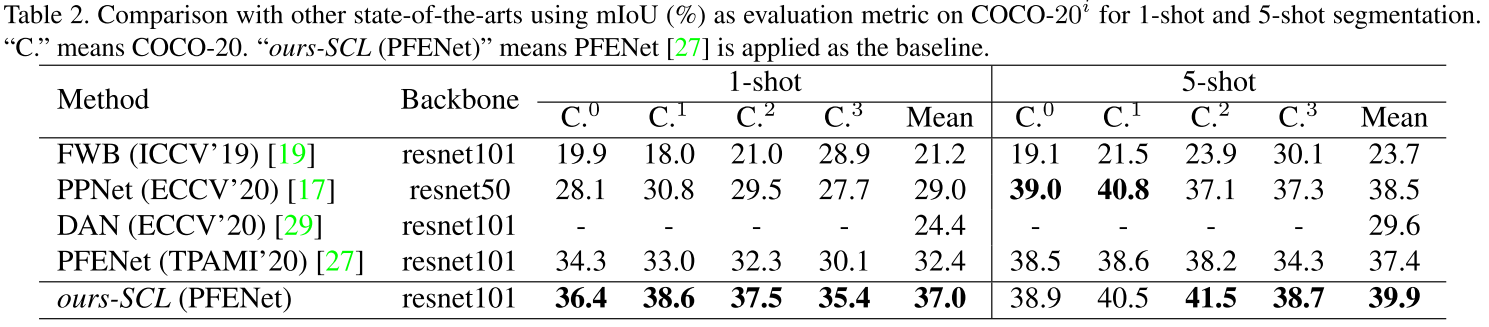### Adaptive Prototype Learning and Allocation for Few-Shot Segmentation

CVPR 2021 | link | github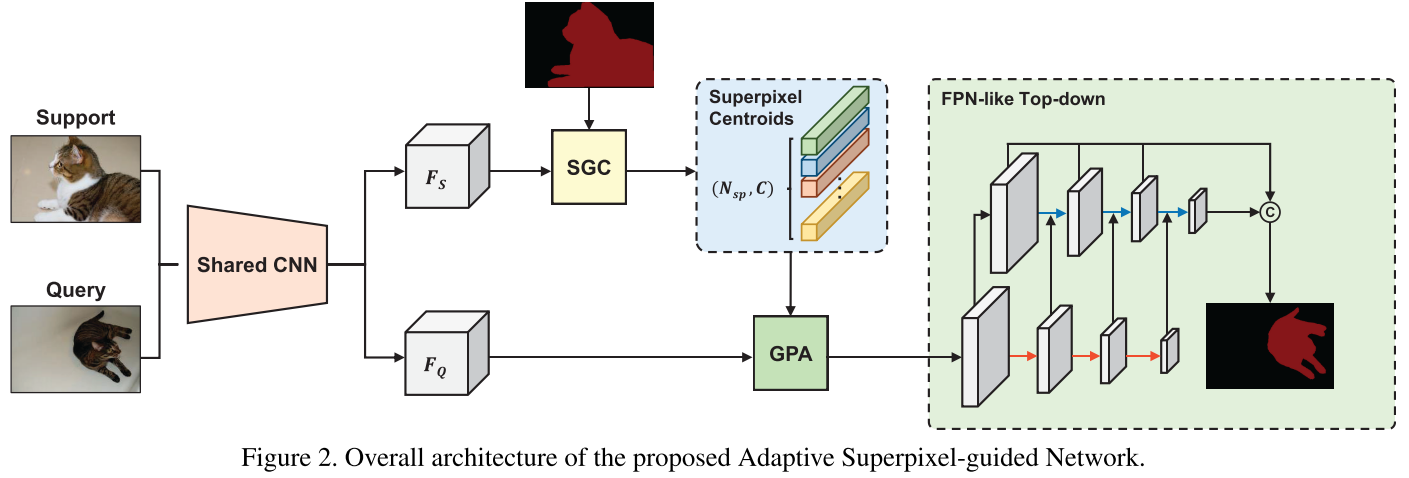Superpixel-guided Clustering：受到 maskSLIC (2016), Superpixel sampling networks (ECCV 2018) 等工作的启发，SGC 模块被用于将特征图用聚类的方式集成到多个 superpixel centroids 中。给定 support 特征 $F_s \in \mathbb{R}^{c \times h \times w}$ 和 support mask $M_s \in \mathbb{R}^{h \times w}$，假设已经获得初始 superpixel 种子，SGC 首先将 support 特征图上每个像素的坐标的值进行放缩后和特征图进行拼接，从而引入位置信息。之后用 support mask 来筛除背景信息，这样我们获得 $F_s^{} \in \mathbb{R}^{(c+2) \times N_m}$，$N_m$ 是在 support mask 中的像素的数量（注意这里我们对变量及其维度表示和原论文略有差别，我在阅读了源码后觉得这样表述会更清晰）。同样，我们也将初始 superpixel 种子的特征和其值放缩后的坐标拼接，有 $S^{0} \in \mathbb{R}^{(c+2) \times N_{sp}}$（$N_{sp}$ 是 superpixel 的数量。获得初始种子的方法见本节最后一段）。接下来，SGC 通过迭代式的方法更新 superpixel-based prototypes：在第 $t$ 轮迭代，首先计算每个像素 $p$ 和所有 superpixels 的 association map $Q^t$：

$$Q^t_{pi} = e^{- || F^{}_p - S^{t-1}_i ||^2}$$

$$S^t_i = \frac{1}{\sum_pQ^t_{pi}} \sum^{N_m}_{p=1} Q^t_{pi} F^{}_p$$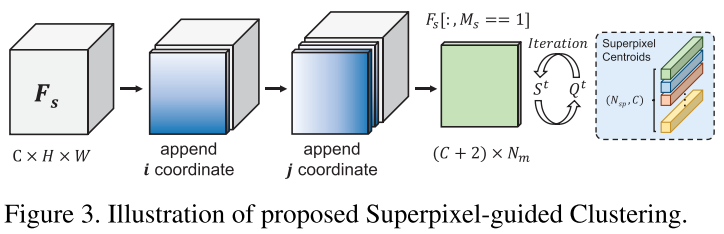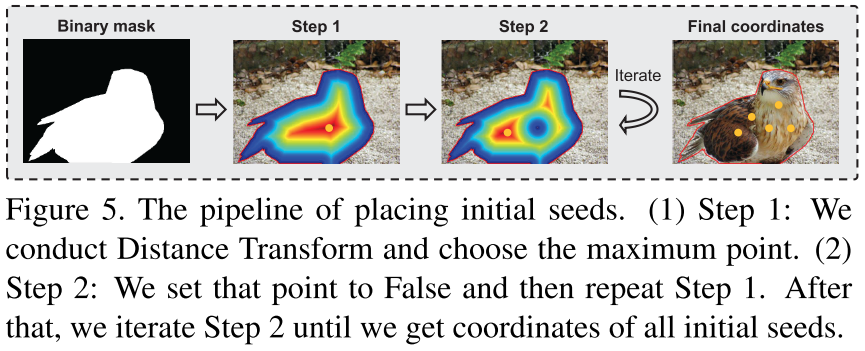Guided Prototype Allocation：首先计算每个 prototype 和 query 特征每个位置的 cosine 相似度 $C^{x,y}_i$，这个相似度信息被输入到一个双分支结构。第一个分支计算每个位置的像素和哪个 prototype 最相似：

$$G^{x,y} = \text{argmax}_{i \in {0, \dots, N_{sp}}} C^{x,y}_i$$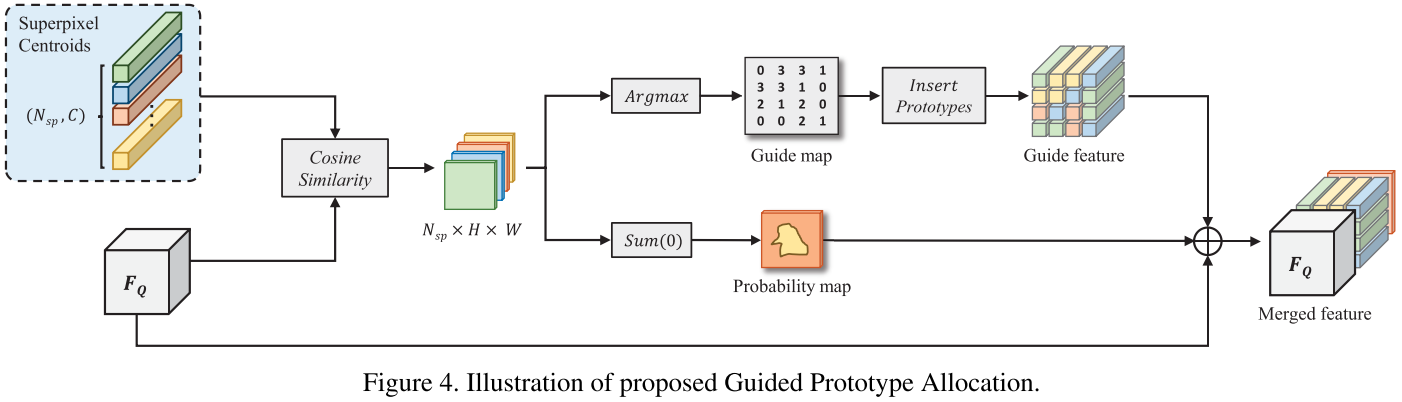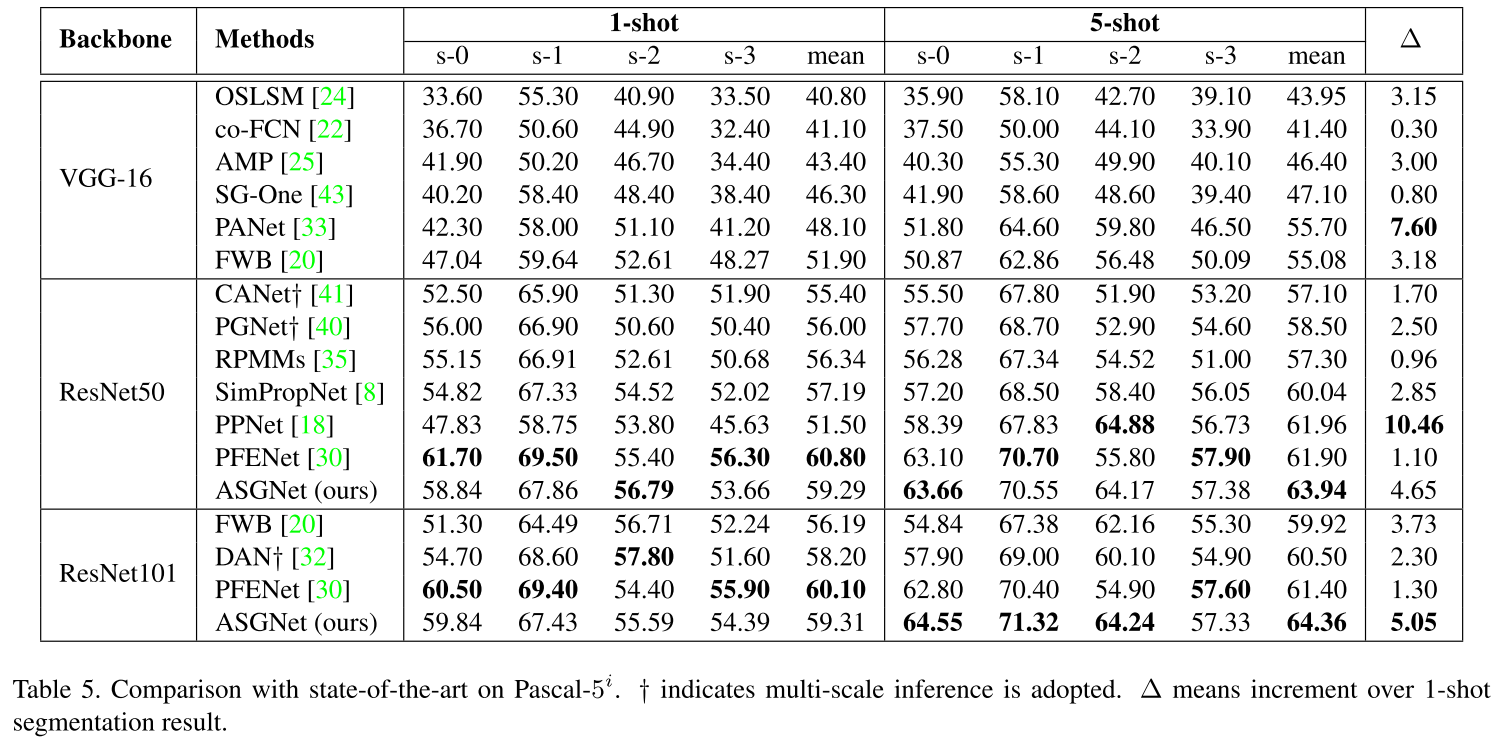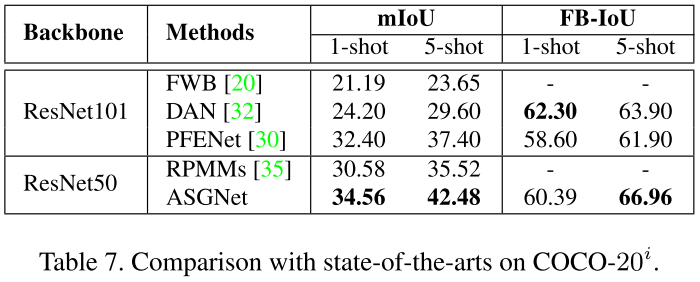### Mining Latent Classes for Few-shot Segmentation

ICCV 2021 | arxiv | github：只给了一个在基础 metric-based 方法上做了些修改的 baseline，没有给论文所提出的方法的代码！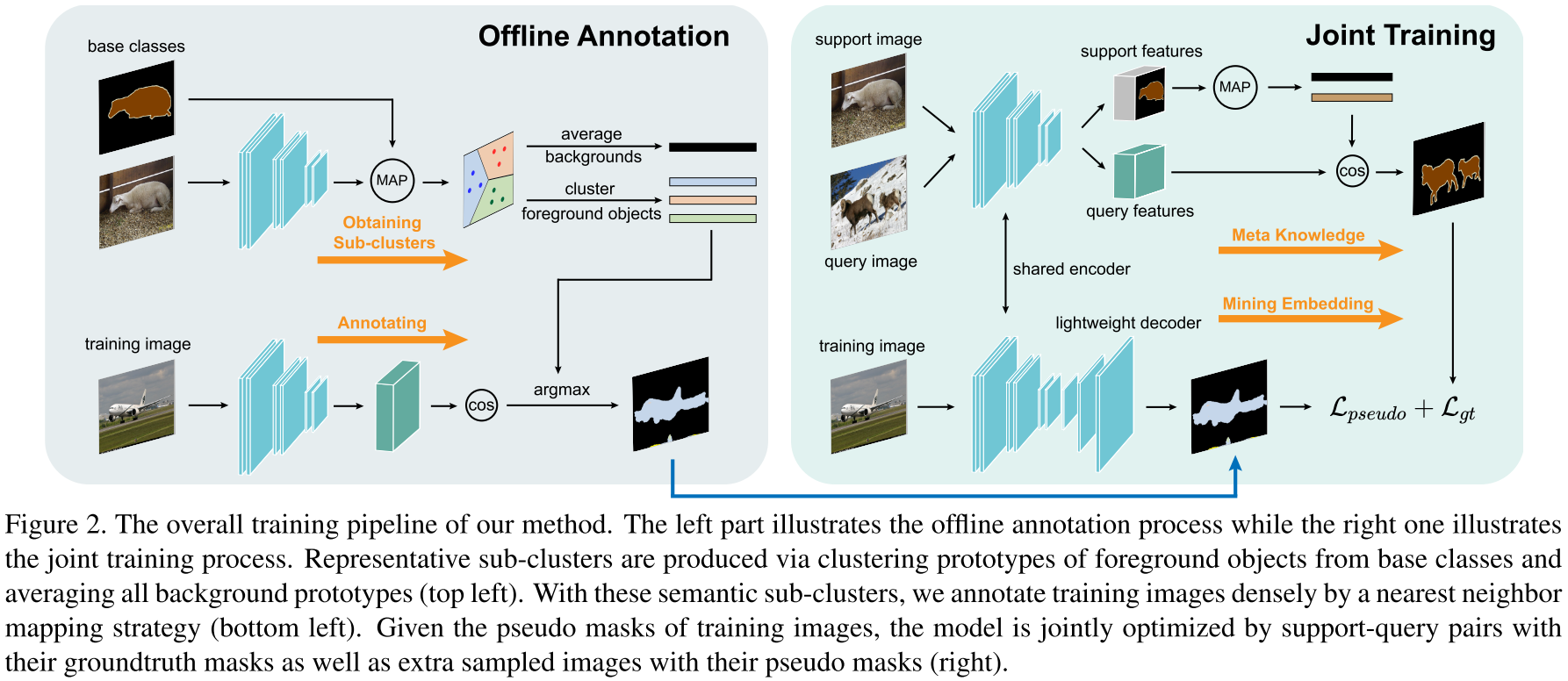1. 将元训练分为离线标注（Offline Annotation）和协同训练（Joint Training）两个阶段。离线标注阶段，模型在所有训练图像得到的所有类别的原型集合（数量上限为图像数 $\times$ 类别数，当然不是每张图像都含所有类别的物体）中通过 K-Means 聚类产生 $K$ 个簇心，同时直接对所有代表背景的原型求均值得到全局背景原型，从而得到 $K+1$ 个新原型，用于给所有训练图像的所有像素用 cosine 相似度打 $K+1$ 类的伪标签（pseudo masks）。除开背景类别外，另外 $K$ 个聚类所得到的类不代表具体的物体种类，而是表示类别的一些共同特征（例如，“马”和“牛”都有的“四足动物”特征）；协同训练阶段采用 episodic training，并额外加了一个分支来用交叉熵训练预测 pseudo masks。该分支由三层带 BN 和 ReLU 的卷积构成，另外考虑到 pseudo masks 的噪声会在训练后期影响性能，因此模型参数更新时计算指数移动平均值（exponential moving average），使模型参数的更新更稳定。
2. 由于学习新类时样本量过少，因此对用于像素二分类的背景和前景原型都分别进行纠偏。背景原型纠偏：在训练阶段采用指数移动平均值来不断用当前的背景原型来更新全局背景原型，在推断时用该全局背景原型和 support 的背景原型线性求和；前景原型纠偏：给定一张 support 图像，根据图像嵌入的 cosine 相似度选择前 N 张相关图像（一个问题就是这里没说相关图像是从哪个集合选），然后根据 support 的前景原型来挑选 K 个最相关的区域原型（这里也没说所谓的“区域”具体是怎么得到的），所有的嵌入通过 ResNet-50/101 的第三层的输出的平均池化得到。之后通过计算前景原型和区域原型的相似度来以加权求和的方式更新前景原型。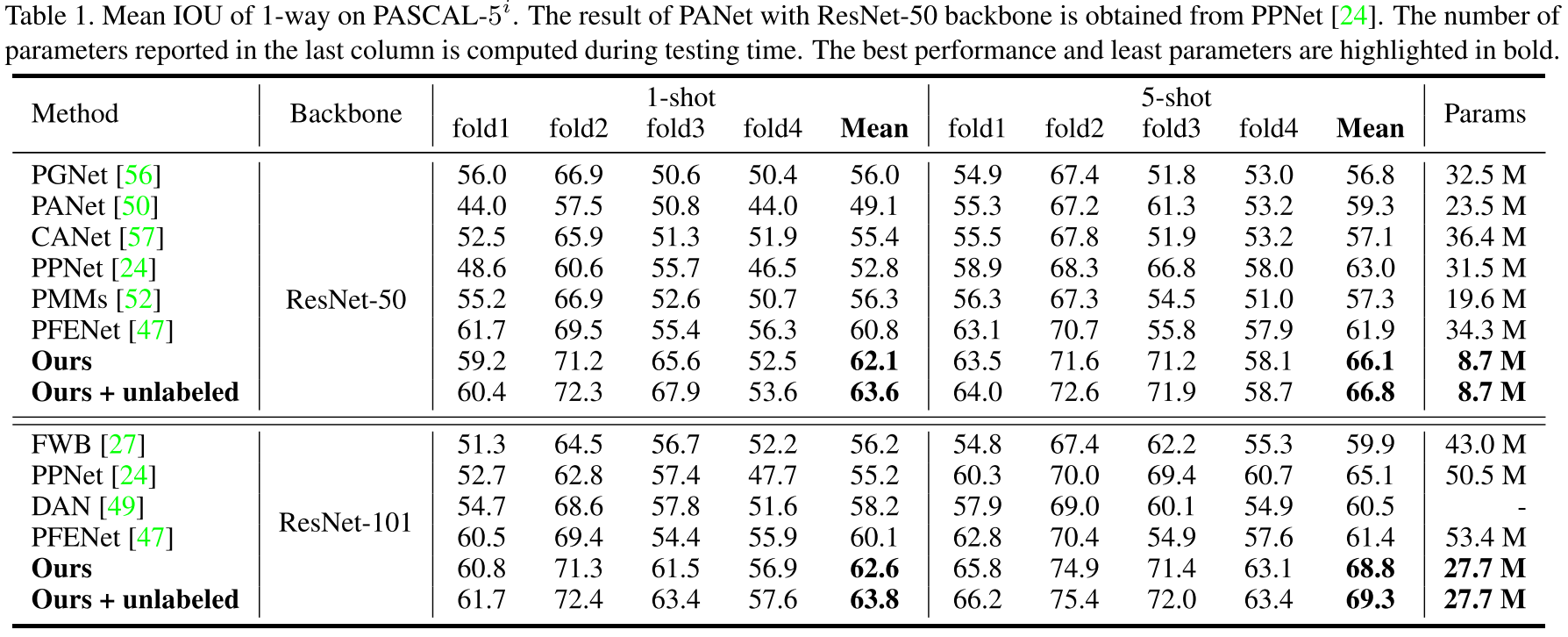### Few-Shot 3D Point Cloud Semantic Segmentation

CVPR 2021 | link | github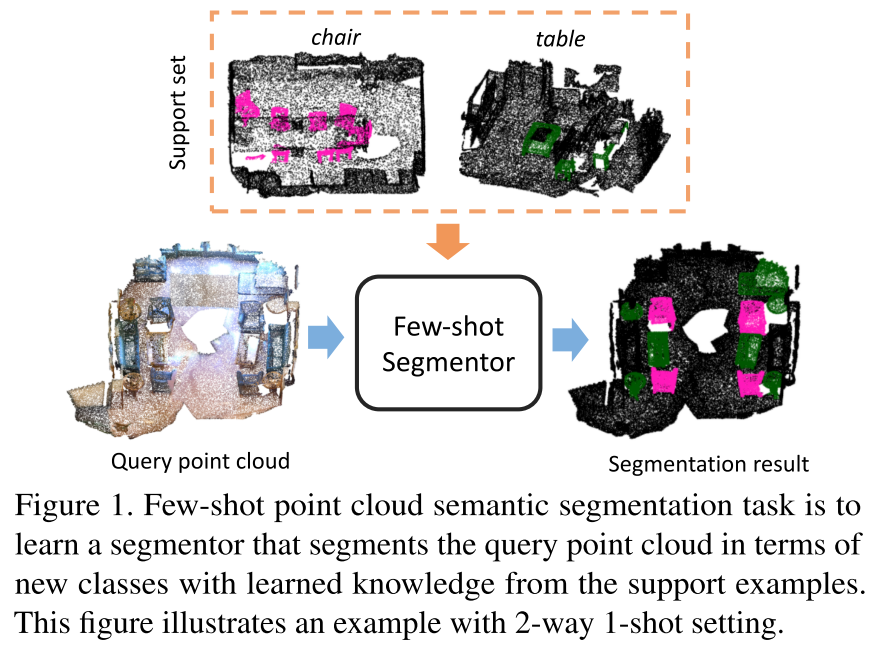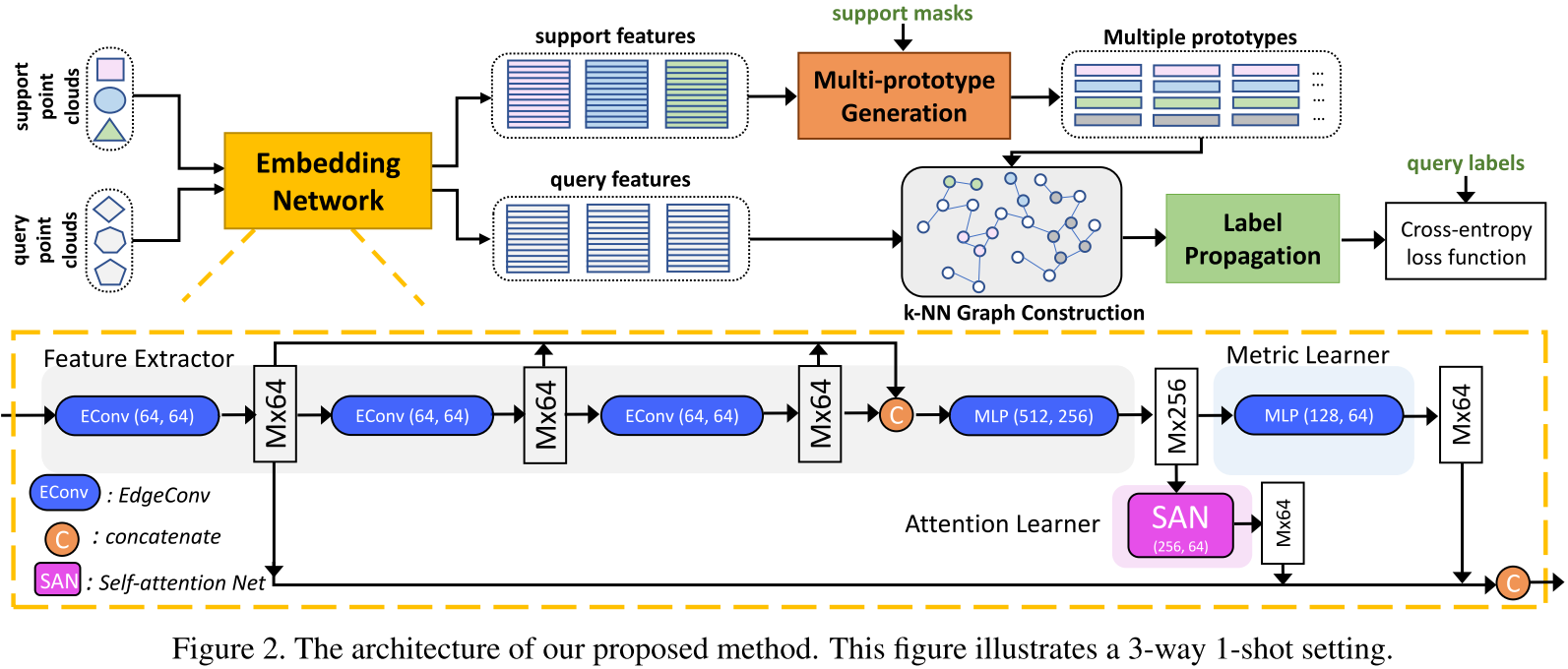embedding network：对标 2D 视觉中的 CNN 等 backbone 网络。论文提出这个网络需要满足 (1) 能够根据局部上下文编码几何结构；(2) 能够根据全局上下文编码点云的语义信息和它们间的语义关系；(3) 能够快速适应不同的小样本任务。因此，本文提出一种 attention-aware multi-level feature learning network 来结合局部几何特征、全局语义特征和 metric-adaptive 特征。具体来说，该网络由三部分组成：(1) feature extractor，选用动态图 CNN 架构的 DGCNN (ACM Trans. Graph. 2019) 来得到局部几何特征（第一个 EdgeConv 层的输出）和语义特征（整个 feature extractor 的输出）；(2) attention learner，选用了 self-attention network (SAN)；(3) metric learner，选用了 MLP 的堆叠并以一个相对更大的学习率更新。

multi-prototype generation：对于 support set 中 $N+1$ 类的每一个，都通过聚类生成 $n$ 个 prototypes。具体地，$n$ 个种子点通过 farthest point sampling 被从 support 点中采样得到。直觉上来说，如果 embedding space 学得够好，这个空间中最远的那些点能够可以内在地表示一个类别的不同视角。之后，我们计算其他点和这些种子点的距离并根据最近邻原则分配，最后计算每个簇的均值作为 prototypes。

transductive inference：该方法首先构建了一张包含 $n \times (N+1)$ 个 prototypes 和 $T \times M$ 个 query 点、总计 $V = n \times (N+1) + T \times M$ 个节点的 k-NN graph。稀疏邻接矩阵 $\mathbf{A} \in \mathbb{R}^{V \times V}$ 通过计算每个点和其 $k$ 个最近邻的高斯相似度得到。为了让邻接矩阵是非负且对称的，有 $\mathbf{W} = \mathbf{A} + \mathbf{A}^{T}$，并进一步进行正则化有 $\mathbf{S} = \mathbf{D}^{-1/2}\mathbf{W}\mathbf{D}^{-1/2}$。同时定义标签矩阵 $\mathbf{Y} \in \mathbb{R}^{V \times (N+1)}$，其中对应有标签 prototypes 的行是 one-hot ground-truth 标签，其余为零向量。给定 $\mathbf{S}$ 和 $\mathbf{Y}$，标签传播有解析解 $\mathbf{Z}^{*} = (\mathbf{I} - \alpha \mathbf{S})^{-1}\mathbf{Y}$。最后每个点云对应的预测通过 softmax 后用交叉熵计算损失。